Question

# Problem 4. Linear Time-Invariant System.s A linear system has the block diagram y(t) z(t) →| Delay...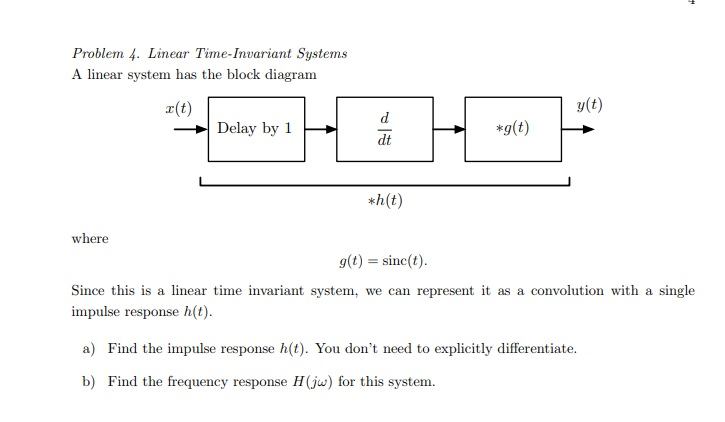Problem 4. Linear Time-Invariant System.s A linear system has the block diagram y(t) z(t) →| Delay by 1 dt *h(t) where g(t) sinc(t Since this is a linear time invariant system, we can represent it as a convolution with a single impulse response h(t) a) Find the impulse response h(t). You don't need to explicitly differentiate. b) Find the frequency response H(j for this system.

We need at least 10 more requests to produce the answer.

0 / 10 have requested this problem solution

The more requests, the faster the answer.

All students who have requested the answer will be notified once they are available.

#### Earn Coins

Coins can be redeemed for fabulous gifts.

Similar Homework Help Questions
• ### 4. A linear time invariant system has the following impulse response: h(t) =2e-at u(t) Use convolution...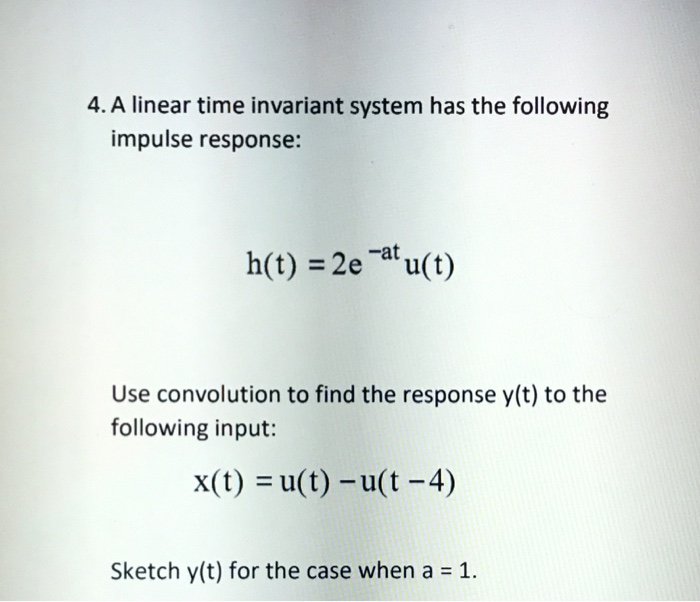4. A linear time invariant system has the following impulse response: h(t) =2e-at u(t) Use convolution to find the response y(t) to the following input: x(t) = u(t)-u(t-4) Sketch y(t) for the case when a = 1

• ### For a continuous time linear time-invariant system, the input-output relation is the following (x(t) the input, y(t) the...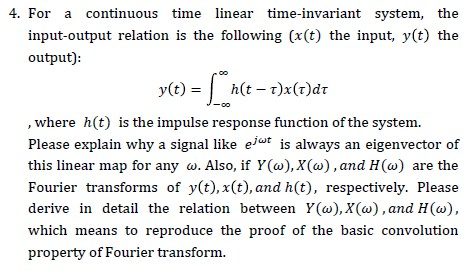For a continuous time linear time-invariant system, the input-output relation is the following (x(t) the input, y(t) the output): , where h(t) is the impulse response function of the system. Please explain why a signal like e/“* is always an eigenvector of this linear map for any w. Also, if ¥(w),X(w),and H(w) are the Fourier transforms of y(t),x(t),and h(t), respectively. Please derive in detail the relation between Y(w),X(w),and H(w), which means to reproduce the proof of the basic convolution property...

• ### 2. Let y(t)(e')u(t) represent the output of a causal, linear and time-invariant continuous-time system with unit...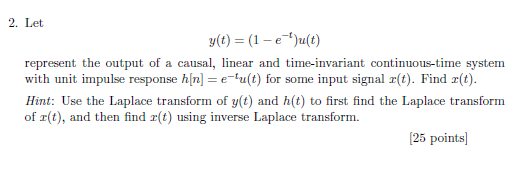2. Let y(t)(e')u(t) represent the output of a causal, linear and time-invariant continuous-time system with unit impulse response h[nu(t) for some input signal z(t). Find r(t) Hint: Use the Laplace transform of y(t) and h(t) to first find the Laplace transform of r(t), and then find r(t) using inverse Laplace transform. 25 points

• ### From homework section of CD: Continuous-time convolution Consider the linear time-invariant system shown below. 7ylt) (t)...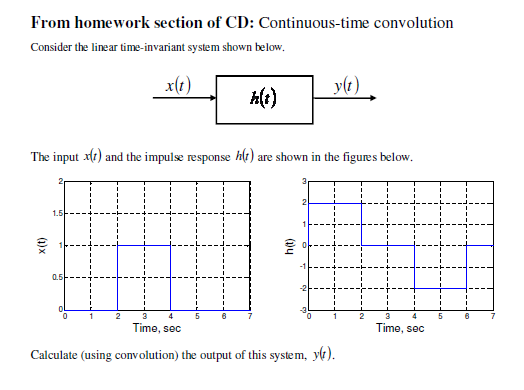From homework section of CD: Continuous-time convolution Consider the linear time-invariant system shown below. 7ylt) (t) The input alt) and the impulse response h(t) are shown in the figures below. ht) Time, sec Time, sec Calculate (using convolution) the output of this system, yo).

• ### 2. Consider a linear time-invariant system with transfer function H(s)Find the (s + α)(s + β) impulse response, h(t), of the system 2. Consider a linear time-invariant system with transfer f...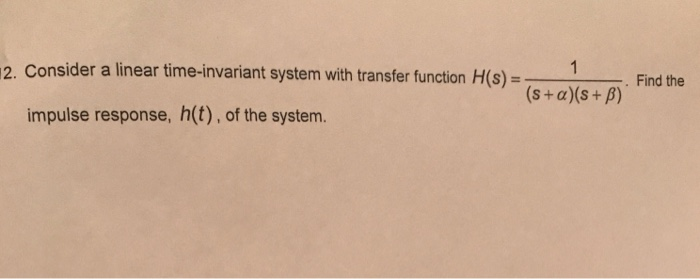2. Consider a linear time-invariant system with transfer function H(s)Find the (s + α)(s + β) impulse response, h(t), of the system 2. Consider a linear time-invariant system with transfer function H(s)Find the (s + α)(s + β) impulse response, h(t), of the system

• ### solve all 22. The input-output relationship for a linear, time-invariant system is described by differential equation...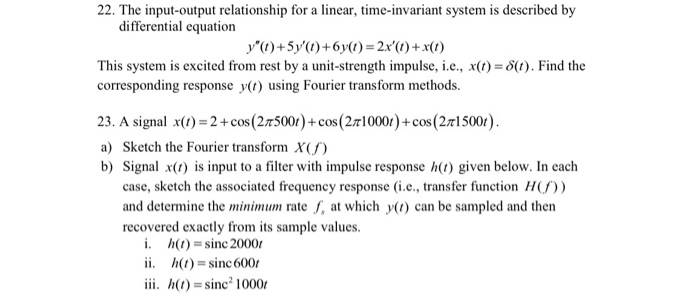solve all 22. The input-output relationship for a linear, time-invariant system is described by differential equation y") +5y'()+6y(1)=2x'()+x(1) This system is excited from rest by a unit-strength impulse, i.e., X(t) = 8(t). Find the corresponding response y(t) using Fourier transform methods. 23. A signal x(1) = 2 + cos (215001)+cos (210001)+cos (2.15001). a) Sketch the Fourier transform X b) Signal x() is input to a filter with impulse response (1) given below. In each case, sketch the associated frequency response...

• ### A linear time invariant system has an impulse response given by h[n] = 2(-0.5)" u[n] –...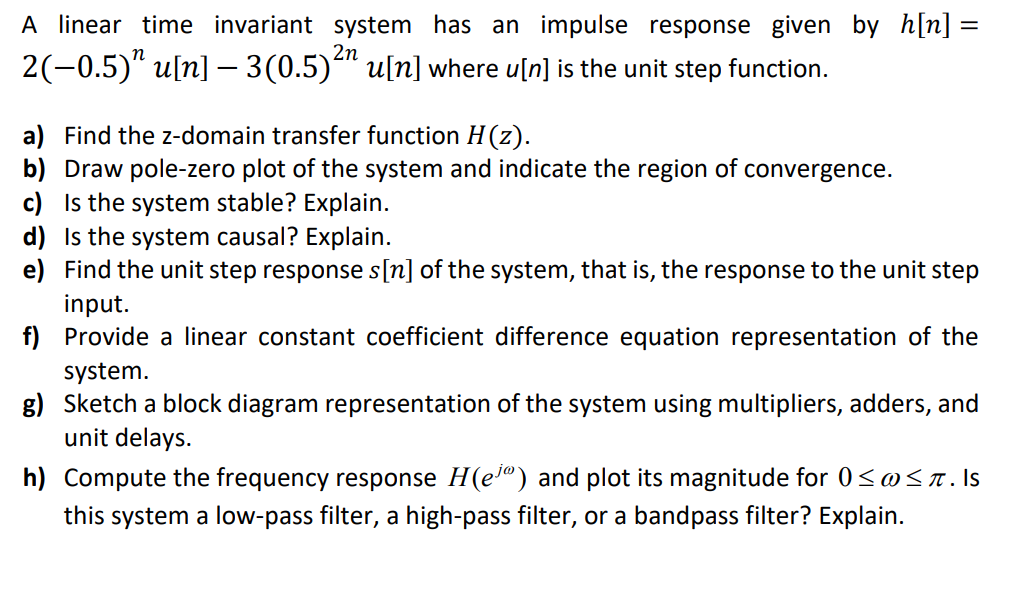A linear time invariant system has an impulse response given by h[n] = 2(-0.5)" u[n] – 3(0.5)2º u[n] where u[n] is the unit step function. a) Find the z-domain transfer function H(2). b) Draw pole-zero plot of the system and indicate the region of convergence. c) is the system stable? Explain. d) is the system causal? Explain. e) Find the unit step response s[n] of the system, that is, the response to the unit step input. f) Provide a linear...

• ### 4- Let the step response of a linear, time-invariant, causal system be (-1).uln] ylnl.ynl-ler uln...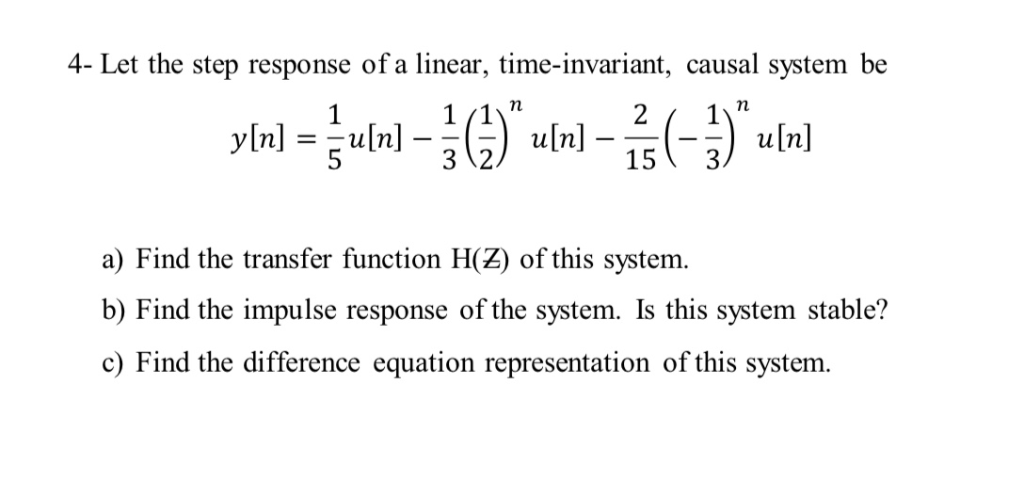4- Let the step response of a linear, time-invariant, causal system be (-1).uln] ylnl.ynl-ler uln].. 15 3 3 12 a) Find the transfer function H(Z) of this system b) Find the impulse response of the system. Is this system stable? c) Find the difference equation representation of this system. 4- Let the step response of a linear, time-invariant, causal system be (-1).uln] ylnl.ynl-ler uln].. 15 3 3 12 a) Find the transfer function H(Z) of this system b) Find the...

• ### The impulse response h(t) of a linear time-invariant system is 2*pi[(t-2)/2]. Find and plot the output...

The impulse response h(t) of a linear time-invariant system is 2*pi[(t-2)/2]. Find and plot the output when the system is driven by an input signal that is identical to the impulse response.

• ### Question 1: (2 marks) Find the zero-input response yz(t) for a linear time-invariant (LTI) system described...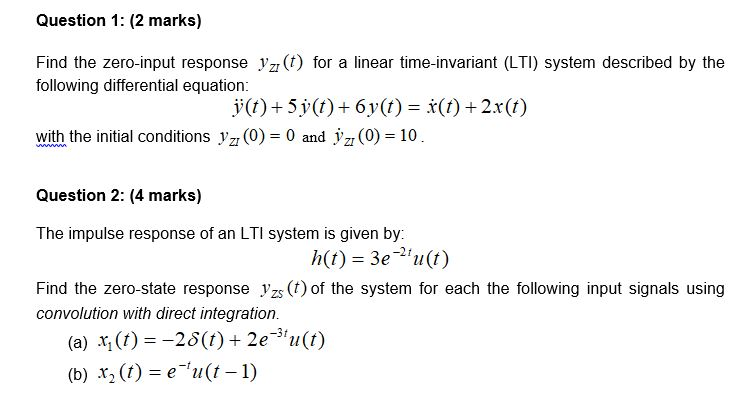Question 1: (2 marks) Find the zero-input response yz(t) for a linear time-invariant (LTI) system described by the following differential equation: j(t) + 5y(t) + 6y(t) = f(t) + 2x(t) with the initial conditions yz (0) = 0 and jz (0) = 10. Question 2: (4 marks) The impulse response of an LTI system is given by: h(t) = 3e?'u(t) Find the zero-state response yzs (t) of the system for each the following input signals using convolution with direct integration....